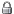LISBON DYNAMICAL SYSTEMS GROUP
 LxDS People Research Seminars Events Opportunities

6th ONE DAY MEETING ON DYNAMICAL SYSTEMS

PROGRAM

 14:00 - 15:00 Diogo Pinheiro (CUNY, USA) Stochastic optimal control under model uncertainty I will discuss stochastic optimal control problems with model uncertainty either in the form of a discrete sequence of random time horizons or in the form of a parametric dependence on a certain switching process. Such problems are interesting not only for their mathematical novelty, but also for their potential application to subjects such as Finance, Actuarial Science, Economics, Population Dynamics and Engineering. I will focus mainly on the derivation of generalized dynamic programming principles, as well as of the corresponding Hamilton-Jacobi-Bellman equations. Time allowing, I will discuss some applications of these abstract results. 15:00 - 16:00 Susana Pinheiro On a stochastic logistic growth model with predation and power-type diffusion coefficient: dynamics and optimal harvesting We consider a logistic growth model with a predation term and a stochastic perturbation given by a diffusive term with power-type coefficient. This SDE has the particularity that the standard conditions for the existence and uniqueness of solutions of SDES (linear growth and Lipschitz) do not hold for a large subset of parameter space. Thus, we start by studying the well posedness of the problem at hand, obtaining a detailed characterization for the existence and uniqueness of solutions. We then provide criteria ensuring extinction and persistence of such population. Additionally, we find subsets of parameter space where (absolutely continuous) stationary measures for the SDE under consideration are guaranteed to exist, providing a description for the corresponding densities. We conclude with an initial application to the optimal management of resources. We consider a real asset such as, for instance, a farm or an aquaculture facility, devoted to the exploration of a unique culture or population whose growth follows a SDE such as described above, and look for the optimal harvesting strategy associated with such culture or population. Break 16:30 - 17:30 João José Gomes (FCUL) Maximum likelihood estimation for linear mixed models A linear model is $Y=X\beta+\varepsilon$ where $Y$, the response vector, is Gaussian $(X\beta,\sigma I)$. A mixed model incorporates two random variables: $B$ the random effects and $Y$ the response vector. In a linear mixed model the unconditional distribution of $B$ and the conditional distribution $(Y| B = b)$ are both Gaussian distributions, $(Y| B =b)\sim N(X\beta+Zb,\sigma I)$ and $B \sim N (0,\Sigma\theta)$. Objective: Parameter estimation by maximum likelihood. Problem: Understand the algorithm.

No registration required

For further informations please send an email to .

VENUE

Complexo Interdisciplinar
Av. Prof. Gama Pinto, 2
1649-003 LISBOA

ORGANIZERS

João Lopes Dias (ISEG, CEMAPRE)
Pedro Duarte (FCUL, CMAF)
José Pedro Gaivão (ISEG, CEMAPRE)

Local information | Links | PrivateThursday, June 20, 2019